## Integration by substitution: the ultimate fudge?

### Integration by substitution: the ultimate fudge?I have often worked with students on the topic of integration by substitution. This isn’t much of a surprise – it’s a fiddly topic with plenty of room for error and, conceptually, it contains challenging ideas. The thing that interests me, though, is that the challenges faced by students on this topic are never the same. In part, this is because there are many different ways of teaching the topic in the first place – and most of them involve some sort of fudge.

If you’re not familiar with the term, a fudge is when something is presented in a vague way to avoid an underlying issue, perhaps because the author feels the truth is too complicated for the reader – but sometimes because the truth is too complicated for the author and they lack the skills to explain it! In maths, a fudge might occur when a student knows where to start and where they should finish, but has to gloss over a step in the middle. Or it may occur when an answer is obtained which is obviously wrong – for example an inequality is pointing in the wrong direction – but finding the source of the error proves too tricky. In these cases, a student might fudge the matter by correcting the answer and hoping that nobody notices the gap in the logic en route.

Another common fudge in mathematics is the abuse of notation, where valid notation is manipulated in a non-standard way in order to obtain a conclusion. Some abuses of notation are straight-up errors, but others are justifiable either as an appropriate shorthand or because they rely on an idea which is true but which is yet to be developed in a particular context. Consider the following example:

Evaluate.

There are many different ways which you may have been taught to solve this – some not involving substitution at all – but the most obvious approach is to use a substitution where. You might therefore write:soand therefore.so.
When. When.

Henceso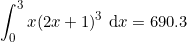And actually, if a student wrote this, I’d be pretty happy. But the first line is problematic. Where did thecome from? And then where did thevanish to? It makes it look likeis a fraction – but most students are taught (correctly) that derivatives aren’t fractions and that you can’t just split them into numerators and denominators… so why make an exception now?

### A formal derivation

For many students, an informal understanding of how this works is enough. But for those looking to pursue mathematics at a higher level, a more thorough understanding can be beneficial.

So: consider functionsandsuch that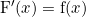, and valuesandsuch that.

Sinceis the derivative of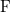, it follows from the fundamental theorem of calculus thatfor any variable.

Specifically, we note that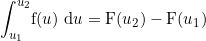.

However, sinceis a function of, we can apply the chain rule to. This shows us that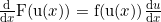.

Applying the fundamental theorem of calculus again, it follows that.

Finally, recall thatand. We can substitute these into the previous result to show that.

We have therefore formed two integrals, each of which is equivalent to. Since they are both equal to the same quantity, the integrals must be equal to one another. Therefore,.

This result is the core of integration by substitution, and students can use it directly should they wish. The formula shows us that a complicated integral (the left-hand side of the equation) can be replaced with a simpler integral (the right hand side). In principle, the simpler integral should be easier to work with. Let’s return to our original example:

Evaluate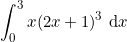.

We again will use the substitution, so. Halving both sides gives us that.

Forming an expression with value 1 is important for the next stage, because 1 is the multiplicative identity.

Therefore,and by the result proved earlier, we can write,

which we can integrate as before to obtain 690.3.

So why do it this way? Is it essential to learn the technique of integration by substitution rigorously, or is it enough to have an informal understanding of why it works? Really, that depends on the student. Whilst some will be perfectly happy to apply methods without fully deriving them, others prefer to know exactly where the rules have come from.

### The product rule

In fact, there are plenty of other techniques and methods that teachers often tend to state when they could instead be derived – many of them  to do with calculus. For instance, the product rule for differentiation, which states that,

can be easily derived if students have already covered implicit differentiation and the differentiation of the natural logarithm – both quite approachable topics. Start by letting, where,andare all functions of. Taking the natural logarithm of both sides enables the product to be written as a sum, and this is the key to finding its derivative, since.

So,and so, differentiating implicitly, we note that.

Multiplying through bygives,

and, recalling thatwe can conclude that,

or, alternatively,.

What are the advantages of learning to derive the formula? It’s certainly not something which most students would ever need to do in an examination. However, it is the case that working through the derivation gives students the opportunity to practice other skills that they do need to master – differentiating implicitly; applying the laws of logarithms; differentiating the logarithmic function – in addition to being introduced to new strategies such as converting a product to a sum. For students going on to tackle more advanced problems in the future, having a broader range of strategies at their disposal will make them more versatile and more likely to succeed in particularly difficult circumstances such as Olympiad or STEP problems.

### The quotient rule

I have also found that students who have become familiar with the idea of deriving formulae for themselves are more likely to look for strategies to derive other results. Take, for example, the quotient rule. Once a student has learnt to derive the product rule, they might consider applying a similar strategy to derive the quotient rule. Starting by letting,

a student might observe thatand so, differentiating implicitly, find similarly that.

Substitutingyields